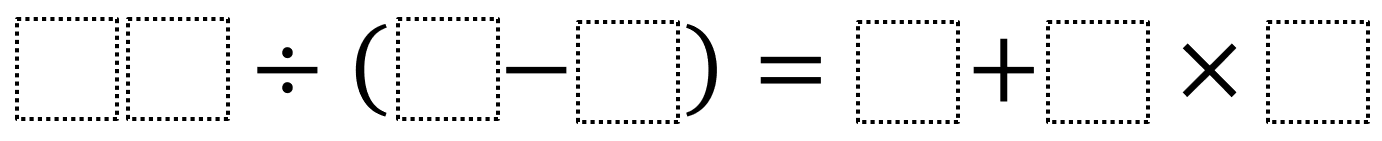# Evaluating Expressions 1

Directions: Using the digits 0 through 9, at most one time each, place a digit in each box to create two true statements: one where the value on each side of the equal sign is greater than 30 and one where it’s less than 30. You may reuse all the digits for each equation.### Hint

How do the order of operations tell you what you should do first? What possible values can you make on the left/right side of the equals sign?

There are many possible answers including 40 ÷ (9 – 7) = 2 + 6 x 3, which has a value of 20 on each side and 50 ÷ (4 – 3) = 2 + 6 x 8, which has a value of 50 on each side.

Source: Robert Kaplinsky

## Add Fractions with Decimal Sums

Directions: Using the digits 1 to 9 at most one time each, place a digit …

1.50 ÷ (4 – 3) = 2 + 6 x 8

2.50 ÷ (4 – 3) = 2 + 6 x 8

3.36 ➗ (5 – 2) = 3 + 9 x 1

4.Lowest:
40 ÷ (9-5) = 3 + 1 x 7

Highest:
60 ÷ (2-1) = 4 + 8 x 7

5.We found that the greatest possible answer is 76 and the smallest possible answer is 3.

Greatest: 76 ÷ (2-1) = 4 + 9 x 8

Smallest: 12 ÷ (8-4) = 3 + 9 x 0

6.Less : 30 divided by (8-5)=4+6×1
More : 90 divided by (4-2)=3+6×7